# JAC Class 10 Maths Solutions Chapter 9 Some Applications of Trigonometry Ex 9.1

Jharkhand Board JAC Class 10 Maths Solutions Chapter 9 Some Applications of Trigonometry Ex 9.1 Textbook Exercise Questions and Answers.

## JAC Board Class 10 Maths Solutions Chapter 9 Some Applications of Trigonometry Exercise 9.1

Question 1.
A circus artist is climbing a 20 m long rope, which is tightly stretched and tied from the top of a vertical pole to the ground. Find the height of the pole, if the angle made by the rope with the ground level is 30°.
Solution :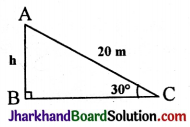sin $$\hat{\mathrm{C}}$$ = $$\frac{AB}{AC}$$
sin 30° = $$\frac{1}{2}$$
$$\frac{1}{2}$$ = $$\frac{AB}{20}$$
AB = $$\frac{20}{2}$$ = 10 mQuestion 2.
A tree breaks due to storm and the broken part bends so that the top of the tree touches the ground making an angle 30° with it. The distance between the foot of the tree to the point where the top touches the ground is 8 m. Find the height of the tree.
Solution :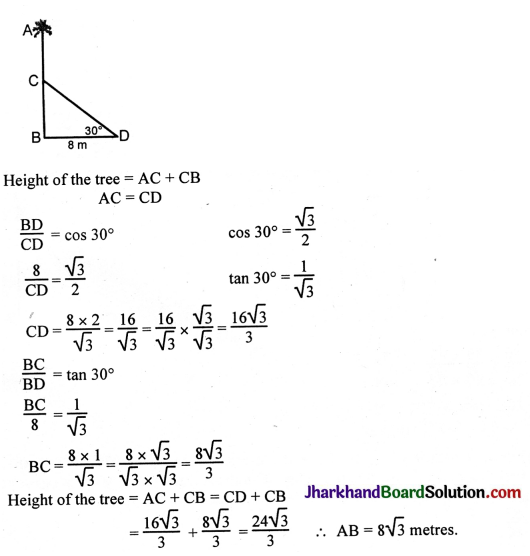Question 3.
A contractor plans to install two slides for the children to play in a park. For the children below the age of 5 years, she prefers to have a slide whose top is at a height of 1.5 m, and is inclined at an angle of 30° to the ground, whereas for elder children, she wants to have a steep slide at a height of 3m, and inclined at an angle of 60° to the ground. What should be the length of the slide in each case?
Solution: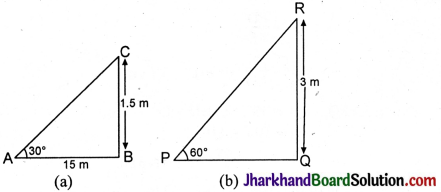(i) Figure (a) shows the slide for children below the age of 5 years.
Let BC = 1.5 m be the height of the slide. Slide AC is inclined at CAB = 30° to the ground.
In right angled ΔABC, sin 30° = $$\frac{BC}{AC}$$
$$\frac{1}{2}=\frac{15}{AC}$$
⇒ AC = 3 m.

(ii) Figure (b) shows the slide for elder children. Let RQ = 3 m be the height of the slide. Slide PR is inclined at ∠RPQ = 60° to the ground.
In right angled ΔPQR, sin 60° = $$\frac{RQ}{PR}$$ ⇒ $$\frac{\sqrt{3}}{2}$$ = $$\frac{3}{PR}$$
PR = $$\frac{3 \times 2}{\sqrt{3}}$$ = 2$$\sqrt{3}$$ m.Question 4.
The angle of elevation of the top of a tower from a point on the ground, which is 30 m away from the foot of the tower, is 30°. Find the height of the tower.
Solution :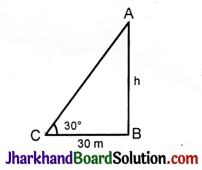tan C = $$\frac{AB}{CB}$$
tan 30° = $$\frac{1}{\sqrt{3}}$$
tan 30° = $$\frac{h}{30}$$
$$\frac{1}{\sqrt{3}}$$ = $$\frac{h}{30}$$
h$$\sqrt{3}$$ = 30
h = $$\frac{30}{\sqrt{3}}=\frac{30 \times \sqrt{3}}{\sqrt{3} \times \sqrt{3}}=\frac{30 \sqrt{3}}{3}$$
h = 10$$\sqrt{3}$$mts.
Hence, height of the tower is 10$$\sqrt{3}$$ mts.

Question 5.
A kite is flying at a height of 60 m above the ground. The string attached to the kite is temporarily tied to a point on the ground. The inclination of the string with the ground is 60°. Find the length of the string, assuming that there is no slack in the string.
Solution :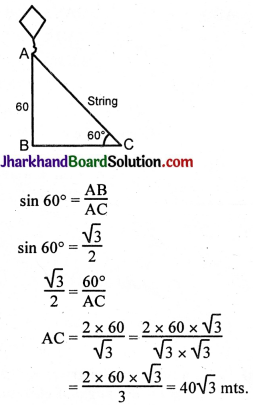Length of the string is 40$$\sqrt{3}$$ mts.Question 6.
A 1.5 m tall boy is standing at some distance from a 30 m tall building. The angle of elevation from his eyes to the top of the building increases from 30° to 60° as he walks towards the building. Find the distance he walked towards the building.
Solution :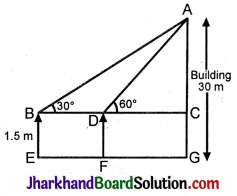Let the boy be standing at point B initially. He walks towards the building and reaches point D. From the figure, the distance walked by the boy towards the building is BD.
AC = AG – CG
AC = 30 – 1.5 = 28.5
Now, in ΔABC, we have
tan 30 = $$\frac{AC}{BC}$$
BC = $$\frac{AC}{tan 30}$$
BC = 28.5$$\sqrt{3}$$
tan 60 = $$\frac{AC}{DC}$$
DC = $$\frac{AC}{tan 60}$$
DC = $$\frac{28.5}{\sqrt{3}}$$
DC = $$\frac{28.5 \sqrt{3}}{3}$$ = 9.5$$\sqrt{3}$$
BD = BC – DC
BD = 28.5$$\sqrt{3}$$ – 9.5$$\sqrt{3}$$
BD = 19$$\sqrt{3}$$
The distance walked by the boy towards the building is 19$$\sqrt{3}$$ m.

Question 7.
From a point on the ground, the angles of elevation of the bottom and the top of a transmission tower fixed at the top of a 20 m high building are 45° and 60° respectively. Find the height of the tower.
Solution :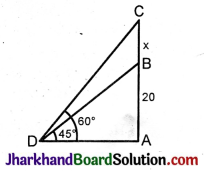tan 60° = $$\sqrt{3}$$
tan 45° = 1
In ΔADC, A$$\hat{\mathrm{D}}$$C = 60
tan 60° = $$\frac{AC}{AD}$$
$$\sqrt{3}$$ = $$\frac{x+20}{DA}$$

In ΔBDA, A$$\hat{\mathrm{D}}$$B = 45°
tan 45° = $$\frac{AB}{AD}$$ = 1
AB = AD = 20 mts.
DA$$\sqrt{3}$$ = x + 20
$$\sqrt{3}$$DA = x + 20
$$\sqrt{3}$$(20) = x + 20
x = 20$$\sqrt{3}$$ – 20
= 20($$\sqrt{3}$$ – 1)
Height of the tower B = 20($$\sqrt{3}$$ – 1) mts.Question 8.
A statue, 1.6 m tall, stands on the top of a pedestal. From a point on the ground, the angle of elevation of the top of the statue is 60° and from the same point the angle of elevation of the top of the pedestal is 45°. Find the height of the pedestal.
Solution :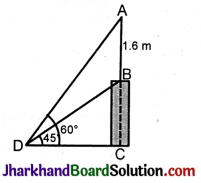tan 60° = $$\sqrt{3}$$
In ΔADC, tan 60° = $$\frac{AC}{CD}$$
$$\sqrt{3}$$ = $$\frac{AC}{CD}$$
AC = $$\sqrt{3}$$CD

In ΔBDC, tan B$$\hat{\mathrm{D}}$$C = tan 45° = $$\frac{BC}{CD}$$
1 = $$\frac{BC}{CD}$$
CD = BC.

A = AC – CB
= AC – CD (∵ CB = CD)
= $$\sqrt{3}$$CD – CD
1.6 = CD($$\sqrt{3}$$ – 1)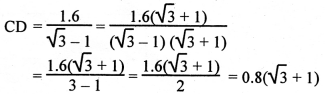Height of the pedestal = 0.8($$\sqrt{3}$$ + 1) mts.

Question 9.
The angle of elevation of the top of a building from the foot of the tower is 30° and the angle of elevation of the top of the tower from the foot of the building is 60°. If the tower is 50 m high, find the height of the building.
Solution :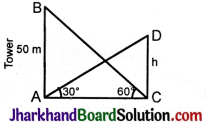Let the height of the building CD be h.
tan 60° = $$\sqrt{3}$$
tan 30° = $$\frac{1}{\sqrt{3}}$$
In ΔABC, tan $$\hat{\mathrm{C}}$$ = $$\frac{AB}{AC}$$
tan 60° = $$\frac{50}{AC}$$
$$\sqrt{3}$$ = $$\frac{50}{AC}$$
AC$$\sqrt{3}$$ = 50°
AC = $$\frac{50}{\sqrt{3}}$$mts.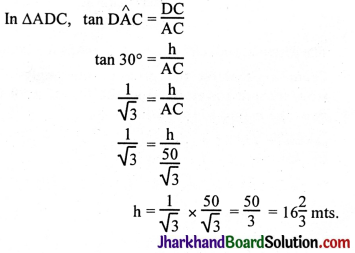Height of the building = 16$$\frac{2}{3}$$ mts.Question 10.
Two poles of equal heights are standing opposite each other on either side of the road, which is 80 m wide. From a point between them on the road, the angles of elevation of the top of the poles are 60° and 30°, respectively. Find the height of the poles and the distances of the point from the poles.
Solution :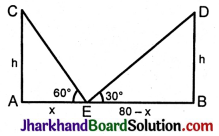Let AE = x.
EB = 80 – x
tan 60° = $$\sqrt{3}$$
tan 30° = $$\frac{1}{\sqrt{3}}$$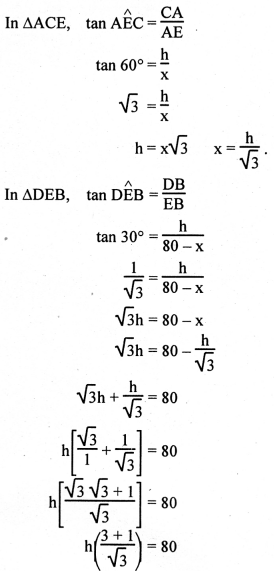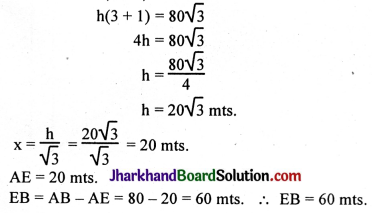Question 11.
A TV tower stands vertically on the bank of a canal. From a point on the other bank directly opposite the tower, the angle of elevation of the top of the tower is 60°. From another point 20 m away from this point on the line joining this point to the foot of the tower, the angle of elevation of the top of the tower is 30°. Find the height of the tower and the width of the canal.
Solution :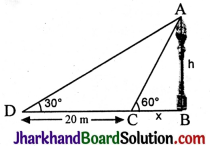tan 60° = $$\sqrt{3}$$
tan 30° = $$\frac{1}{\sqrt{3}}$$
In ΔABC, tan 60° = $$\frac{AB}{BC}$$ = $$\frac{h}{x}$$
$$\frac{h}{x}$$ = $$\sqrt{3}$$
∴ h = $$\sqrt{3}$$x
In ΔADB, tan 30° = $$\frac{AB}{DB}$$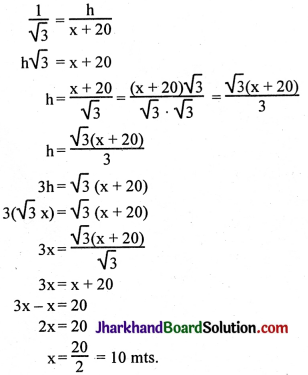Height = x$$\sqrt{3}$$ = 10$$\sqrt{3}$$ mts. Width of the canal = 10 mts.Question 12.
From the top of a 7 m high building, the angle of elevation of the top of a cable tower is 60° and the angle of depression of its foot is 45°. Determine the height of the tower.
Solution :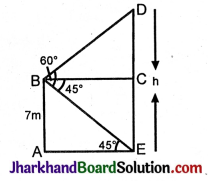In ΔDBC, tan 60° = $$\frac{DC}{BC}$$
$$\sqrt{3}$$ = $$\frac{DC}{BC}$$
= $$\frac{DC}{AE}$$ = $$\frac{DC}{7}$$ (BC = AE).

In ΔABE, tan 45° = $$\frac{AB}{AE}$$
1 = $$\frac{7}{AE}$$
∴ AE = 7 mts.
AB = BC = 7 mts.
DC = 7$$\sqrt{3}$$
∴ DE = DC + CE
= 7$$\sqrt{3}$$ + 7 = 7($$\sqrt{3}$$ + 1) mts.

Question 13.
As observed from the top of a 75 m high lighthouse from the sea-level, the angles of depression of two ships are 30° and 45°. If one ship is exactly behind the other on the same side of the lighthouse, find the distance between the two ships.
Solution :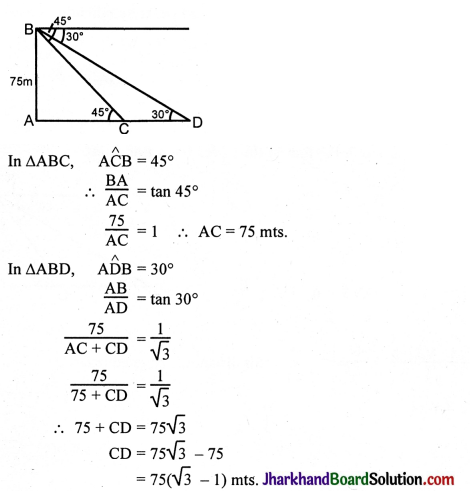Hence, the distance between the two ships is 75($$\sqrt{3}$$ – 1) m.Question 14.
A 1.2 m tall girl spots a balloon moving with the wind in a horizontal line at a height of 88.2 m from the ground. The angle of elevation of the balloon from the eyes of the girl at any instant is 60°. After some time, the angle of elevation reduces to 30°. Find the distance travelled by the balloon during the interval.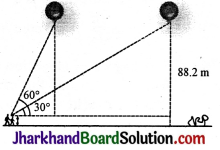Solution :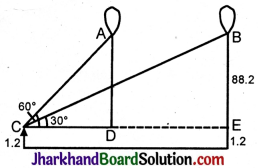Let the initial position of the balloon be A and final position be B.
Height of the balloon above the girl’s height = 88.2 m – 1.2 m = 87m
Distance travelled by the balloon = DE = CE – CD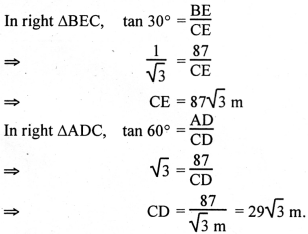Distance travelled by the balloon, DE = CE – CD
= (87$$\sqrt{3}$$ – 29$$\sqrt{3}$$) m
= 29$$\sqrt{3}$$ (3 – 1) m
= 58$$\sqrt{3}$$ m.

Question 15.
A straight highway leads to the foot of a tower. A man standing at the top of the tower observes a car at an angle of depression of 30°, which is approaching the foot of the tower with a uniform speed. Six seconds later, the angle of depression of the car is found to be 60°. Find the time taken by the car to reach the foot of the tower from this point.
Solution :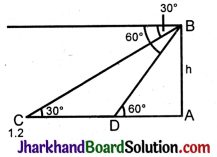In ΔBCA, tan 30° = $$\frac{h}{CA}$$
$$\frac{1}{\sqrt{3}}$$ = $$\frac{h}{CA}$$
CA = h$$\sqrt{3}$$ mts.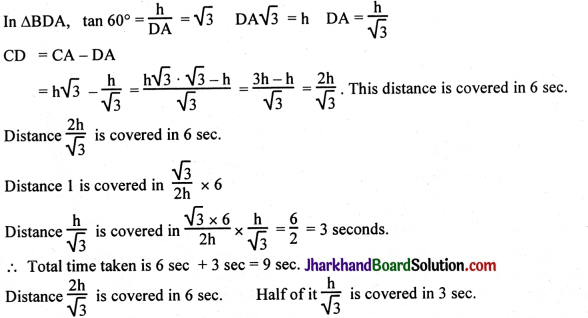Question 16.
The angles of elevation of the top of a tower from two points at a distance of 4 m and 9 m from the base of the tower and in the same straight line with it are complementary. Prove that the height of the tower is 6 m.
Solution :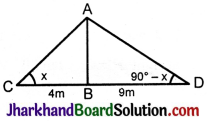Let AB be the tower. ∠ABC = x
∴ ∠ADB = 90° – x
In ΔABC tan x = $$\frac{AB}{BC}$$
tan x = $$\frac{AB}{4}$$ ……………(i)
In ΔADB tan (90° – x) = $$\frac{AB}{9}$$
cot x = $$\frac{AB}{9}$$ ……………(ii)
(i) × (ii)
tan x × cot x = $$\frac{AB}{4}$$ × $$\frac{AB}{9}$$
tan x × $$\frac{1}{tan x}$$ = $$\frac{\mathrm{AB}^2}{36}$$
1 = $$\frac{\mathrm{AB}^2}{36}$$
AB² = 36
AB = ± $$\sqrt{36}$$
AB = ± 6
∴ Height of the tower AB = 6 m.
Note: C and D can be taken on the same side of AB.